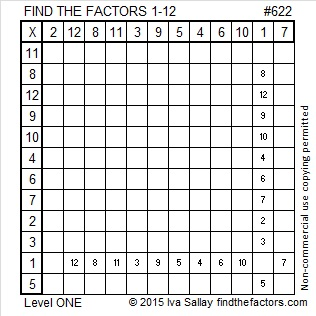# 622 and Level 1

Can 622 be written as the sum of consecutive numbers?

622 is greater than 6 and is divisible by 2, but not by 4, thus it can be expressed as the sum of 4 consecutive numbers:

154 + 155 + 156 + 157 = 622

622  is the sum of the sixteen prime numbers from 11 to 71.Print the puzzles or type the solution on this excel file: 12 Factors 2015-09-21

—————————————————————————————————

• 622 is a composite number.
• Prime factorization: 622 = 2 x 311
• The exponents in the prime factorization are 1 and 1. Adding one to each and multiplying we get (1 + 1)(1 + 1) = 2 x 2 = 4. Therefore 622 has exactly 4 factors.
• Factors of 622: 1, 2, 311, 622
• Factor pairs: 622 = 1 x 622 or 2 x 311
• 622 has no square factors that allow its square root to be simplified. √622 ≈ 24.9399.—————————————————————————————————This site uses Akismet to reduce spam. Learn how your comment data is processed.